﻿ Black Hole Entropy is Quantum Intrinsic EntropyPublications are Open
Access in this journal
Article Versions
Export Article
• Normal Style
• MLA Style
• APA Style
• Chicago Style
Original Article
Open Access Peer-reviewed

### Black Hole Entropy is Quantum Intrinsic Entropy

Xiao Lin Li
International Journal of Physics. 2022, 10(1), 64-69. DOI: 10.12691/ijp-10-1-5
Received January 01, 2022; Revised January 29, 2022; Accepted February 07, 2022

### Abstract

The author proposes a new black hole model. The particles only satisfying the special merging condition can merge together to form a black hole. The particles have intrinsic entropy in quantum mechanics. The particles arrange together by a special distribution to form a special thermodynamic system. The special thermodynamic system is just the black hole. The black hole entropy is just the sum of the intrinsic entropy of these particles merging to form the black hole. The black hole entropy is a kind of intrinsic entropy in quantum mechanics, and the black hole temperature is a kind of intrinsic temperature in quantum mechanics. The special distribution is named by black hole distribution. The black hole can be seen as a special quantum condensation system which be named by black hole condensation. The merging condition has several different physical meanings. It is an equal temperature condition, and it is a correspondence condition also. The particles have a new property named by correspondence radius. We can derive out the Verlinde entropy gravity proposal formula from this black hole model. And we can prove that the Verlinde entropy gravity proposal formula only hold true in the process of black hole merging. The Verlinde entropy gravity theory is not correct universally.

### 1. Introduction

The micro statistical origin of the black hole entropy have been a mystery since Bekenstein and Hawking proposed black hole entropy 1, 2. The author proposed a new gravity theory in previous paper 3. It is the Planck Gravitation Theory, or the Planck Gravity Theory. In the Planck Gravity Theory, there exist a black hole model. This black hole model is just a simple micro model, and this model happens to be able to provide a simple micro statistical origin about the black hole entropy.

A outside particle merging into a small black hole to form a bigger black hole 4. It is Figure 1.

The outside particle has rest mass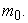The small black hole has mass M. The radius of the small black hole is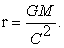The outside particle must meet a special merging condition. The condition is the formula (1.1).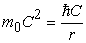(1.1)

With the continuous cycle of this merging process, these particles form a special thermodynamic system. The entropy of this special thermodynamic system is just the black hole entropy. The entropy of the system satisfies the formula (1.2).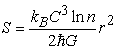(1.2)

The number n is the intrinsic degrees of freedom of the outside particle. It is not the degrees of freedom of vibration, and it is not the degrees of freedom of movement. Please distinguish it carefully. For example, the outside particle has spin property. Its spin is 1, or its spin is 1/2. So the particle has two possible microscopic states. So its intrinsic degree of freedom is 2. To a particle, its spin is a constant.

We identify the constant of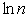by a new constant D. It is formula below.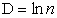So we get a simplified formula (1.3).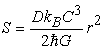(1.3)

There is only one constant difference between (1.3) and the Hawking entropy formula of black hole 2.

About the detailed description of the black hole merging model, please refer to the previous paper 4.

In the previous paper 4, the author described such a black hole model, but it is not clear enough. Therefore, the author continues to explain the black hole model in detail in this paper. In the following explanation, we will clearly see that the black hole entropy is a pure property of quantum mechanics. And we will find an unintentional correlation between this black hole model and the Verlinde entropy gravity formula 5. From this black hole mode, we will find that the Verlinde entropy gravity theory is not correct universally.

### 2. Black Hole Entropy is Intrinsic Entropy

2.1. Intrinsic Entropy

Firstly, let’s inspect the intrinsic degrees of freedom of the outside particle. For example, to the spin property of particles, the number of intrinsic degrees of freedom is two, so n=2. So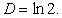Obviously, the particle has other intrinsic degrees of freedom also. If the particle has another intrinsic degree of freedom, for example the number of another intrinsic degree is m, so the intrinsic degrees of freedom of the particle is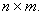So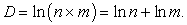The degree of freedom is intrinsic property of the particle. These intrinsic degrees of freedom are originated in quantum mechanics, and it is a pure quantum property. It is not related to the motion of particles. And it is not associated with the vibration of particle. As long as the particle exist, it has these intrinsic degrees of freedom. The intrinsic degrees of freedom correspond to the microscopic states of particle. The microscopic states of particle correspond to the entropy of particle. It is means that the particle has a new kind of quantum properties. It is intrinsic entropy of particle. The value of intrinsic entropy of particle is formula (2.1). The number n is the intrinsic degrees of particle. This is the standard statistical mechanics result 6, 7.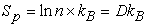(2.1)

In previous paper 4, the author derived out a result. In the merging process, the total numbers of particles of the black hole are formula (2.2).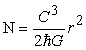(2.2)

So we can get that the total entropy of the particles in the black hole are formula (2.3).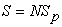(2.3)

And the temperature formula of the black hole is (2.4).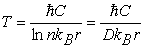(2.4)

This is means that the black hole entropy is the sum of intrinsic entropy of particles merging the black hole. So the black hole entropy is a kind of intrinsic entropy also. The black hole is a special thermodynamic system. The black hole entropy is the intrinsic entropy of the special thermodynamic system. So the black hole entropy is a pure quantum property. It is not associated with classical thermodynamics at all.

Quantum mechanics brings a new kind of entropy, that is intrinsic entropy. The black hole entropy is just one of them. Perhaps there exist other kind of intrinsic entropy. This topic is well worth studying.

2.2. Equal Temperature Condition

Secondly, let’s inspect the merging condition (1.1). Its essence is an equal temperature condition.

In classical thermodynamics, to the particles, there exist a formula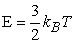6, 7. But it is invalid in merging process of black hole. To the intrinsic entropy of particle, it no longer applies.

In merging process of black hole, to the intrinsic entropy of particle, the valid formula is (2.5). E is the energy of the outside particle merged black hole. Formula (2.5) is in line with the laws of thermodynamics.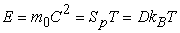(2.5)

So, we can see. The energy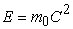can be seen as intrinsic energy of particle. The temperature T can be seen as intrinsic temperature of particle. In this case, the energy, the entropy, the temperature, the three physical quantities can be seen as the intrinsic properties of particle.

The temperature of the black hole is (2.6).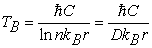(2.6)

Take (1.1) and (2.5) into (2.6), so we get the result.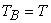The temperature of particle is equal with the temperature of the black hole.

So we can get a result. To the special merging condition (1.1), its essence is an equal temperature condition. Only the intrinsic temperature of particle is equal with the temperature of the black hole, the particle can merge with the black hole to form a bigger black hole. Once we understand this, we can see that it is an obvious condition.

Let's assume particle A. If its temperature is less than the temperature of the black hole, when particle A merge with the black hole, according to the laws of thermodynamics, the heat will transfer from black hole to A. This clearly conflicts with the definition of the black hole. According to the definition of a black hole, the heat can’t flow from black hole to A.

Let's assume particle B. If its temperature is more than the temperature of the black hole, when particle B merge with the black hole, according to the laws of thermodynamics, the heat will transfer from B to black hole. This will cause the black hole to rise in temperature. This will cause that the temperature of the merged bigger black hole is higher. This conflicts with the temperature formula of the black hole.

In fact, only the combination of two equal-temperature objects can satisfy both conditions. The first condition is that must satisfy the laws of thermodynamics. Another condition is that must satisfy the definition of the black hole.

2.3. Correspondence Condition

In previous paper 4, to a particle which have rest mass, there exist a relationship between its Compton wavelength and its black hole radius. The product of these two quantities is the Planck length 8, 9. That is formula (2.7).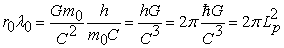(2.7)

The Compton wavelength of the particle is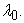The radius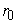can be seen as an intrinsic property of the particle. Any particles with rest mass have this intrinsic radius. Any particles with rest mass have the corresponding intrinsic radius. We call the corresponding intrinsic radius by correspondence radius below. Please remember this concept.

Let’s inspect the merging condition formula (1.1). We change its form. Here R is the radius of the black hole.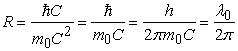The radius of the black hole which the particle will merge into is just the Compton wavelength of the particle.

So we get formula (2.8).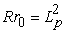(2.8)

It is means that the correspondence radiusof the particle is just the corresponding length of the black hole radius R. So the merging condition is a corresponding condition. Only particle which satisfy this corresponding condition can merge with the black hole to form a bigger black hole. This is a very surprising result.

The merge process is constantly looping, and the radius R of the merged black hole is getting larger and larger, and the correspondence radiusis getting smaller and smaller.

From a certain perspective, this merge process can be seen as a merging process of correspondence radiusof the particles. This perspective is easier to understand. So the radius of the black hole is sum of the correspondence radiusof particles. So we can calculate the total number of particles merged into the black hole.

From formula (2.8), we can get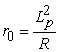So the total number of particles merged into the black hole is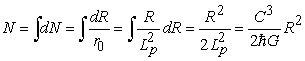(2.9)

This merging process is represented by Figure 2.

But because gravity has linear synthetic properties, and the particles have the wave properties in quantum mechanics, so the more accurate representation is Figure 3. The merging process can be seen as the shells merging process.

This perspective is easier to understand. But we must distinguish clearly. The particle is not a true black hole, it just has the correspondence radius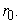It is a property brought about by gravity. Any particles with rest mass have this property of the correspondence radius. The particle entropy is the formula (2.1), but is not the black hole entropy formula (1.3). Only the particles system formed by the merge process are the true black hole which its entropy satisfies the formula (1.3). Please distinguish it clearly.

From (2.1), each particle has the intrinsic entropy.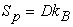So the entropy of the system formed by these particles is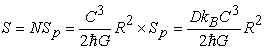(2.10)

The entropy formula of the system is same as the black hole entropy formula. There is only one constant different from the Hawking black hole entropy formula 2. So the particles system is indeed a black hole. So the black hole entropy is the sum of intrinsic entropy of particles merging to form the black hole.

So the energy of the black hole is the total energy of all particles. And the particle energy satisfies the merging condition (1.1). So can get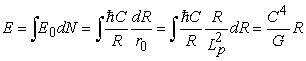(2.11)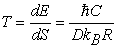(2.12)

In this black hole model, the three formulas can be easily deduced.

Let’s inspect the formula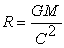again. The formula is in the Planck Gravity Theory. From this formula, we get the formula (2.7). The formula (2.7) is a correspondence property of the particle. But to a single particle, it is not a real black hole. To a single particle, it does not have the black hole entropy. Entropy is associated with the microstates. But to a single particle, if the particle has the entropy property named by black hole entropy, we can't find the associated microstates with the entropy. To a single particle, we can only find the intrinsic microstates. So we can’t assume that a single particle has the properties of black hole entropy. So, to a single particle, we can’t say that the radiusis the black hole radius of the particle. The exact statement is that the radiusis the correspondence radius of particle. Please distinguish carefully. Only the particles system can be seen as the black hole, and the system has the entropy property.

But the total energy of the black hole is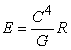. So its equivalent rest mass is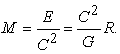So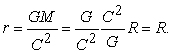So we can see. To a black hole system, the radius calculated by formula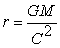is indeed the radius of the black hole.

So, to a single particle, and to a black hole system, although they have same formula, but have different physical meanings.

From the black hole model above, we can get a result easily. The center particle of a black hole is a special particle. Its rest mass of the particle is just the Planck Mass, and its correspondence radius is just the Planck Length. Only the particle which rest mass is the Planck Mass can become the seed at the center of a black hole. This is another physical meaning about the Planck Length. When the rest mass of a particle is equal to the Planck Mass, the particle does not become a black hole, but the particle become the seed of a black hole. This is a whole new perspective. A single particle can’t become a black hole, and the entropy of the particle is not black hole entropy. To a single particle, it only has the quantum intrinsic entropy. Only a system formed by many particles can become a black hole, and the entropy of the system is black hole entropy. This is fully in line with thermodynamic principles.

### 3. Black Hole is System of Quantum Mechanics

Through the description above, we get the result. The black hole system is a special thermodynamic system. And the black hole is a complete system of quantum mechanics. Its entropy, energy and temperature are intrinsic properties of quantum mechanics. Because the intrinsic properties only exist in quantum mechanics, not exist in classical physics.

From the perspective of quantum mechanics, we can understand by this way. The particle be in the gravitation field of the black hole. And the state of the particle is a quantum state. Only the black hole and the particle satisfy the merging condition, the particle can be in the base state of the gravitation field of the black hole. Only the particle in the base state can merge with the black hole to form a bigger black hole. The merging condition is a base state condition also. This is an obvious result in fact.

Why the particles in black hole only have the intrinsic degrees of freedom? This is also easy to understand in quantum mechanics. Because the particle is in base state of quantum mechanics, its degrees of freedom of movement are completely limited. The particle lost its degrees of freedom of movement completely. So the particle only has the intrinsic degrees of freedom. This is entirely a quantum mechanics phenomenon. Only from the perspective of quantum mechanics, we can really understand the black hole, and we can really understand the black hole entropy. The black hole is a complete system of quantum mechanics. To the black hole, entropy, energy and temperature, all of them are intrinsic properties of quantum mechanics.

Please distinguish carefully. The particles do not have degrees of freedom of movement. This state does not mean that the particles are completely motionless. This is a state of quantum mechanics completely. In classical physics, we can't understand this phenomenon correctly. If particles do not have degrees of freedom of movement in classical physics, particles are completely stationary and do not move. But in quantum mechanics, the degrees of freedom are property of quantum state. In quantum mechanics, particles move even if they do not have degrees of freedom of movement. To the conception about the degrees of freedom of movement, please distinguish carefully between quantum mechanics and classical physics. For example, in bose statistics 6, if all particles are in the base state, the degrees of freedom of movement of these particles are zero, but it does not mean these particles are completely stationary. These particles are still moving.

Through the description above, we can get a result. The black hole merged by many particles has a special structure. This special structure can be seen as a special distribution mode. We name it by black hole distribution. The black hole distribution can be represented by Figure 2 or Figure 3. The particles arranged one by one. There are no jumps in the middle, and no gaps. The particles do not have degrees of freedom of movement, but only have intrinsic degrees of freedom. This special distribution represents a kind of special quantum thermodynamic system. The black hole system can be seen as a kind of quantum condensation system. We name it by black hole condensation. The black hole condensation can be seen as the base state of a quantum gravity system. This is similar to the Bose condensation system. There exists some similarity between gravity system and thermodynamic systems. This topic is well worth studying.

Because all particles do not have degrees of freedom of movement, but only have intrinsic degrees of freedom. To a particles system, if the number of particles remains fixed, the minimum of the entropy of the particles system is the black hole entropy obviously. When these particles form a black hole, the entropy of the particles system is minimum. From holographic principle 10, if the radius of the space is fixed, the black hole entropy is maximum. There seems to be a correspondence between the two situations. This topic is well worth studying.

Because the black hole is a system of quantum mechanics, so the particles in black hole have the probability of jumping out of the black hole. This is also the result of the probability of quantum mechanics. This is also the essence of the black hole radiation, and this is also the essence of the black hole temperature.

### 4. Discuss the Verlinde Entropy Gravity Proposal Formula

Because the particle satisfies the merging condition. That is formula (4.1).(4.1)

And the temperature of particle is formula (4.2). The r is the radius of the black hole.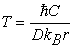(4.2)

About the entropy of the particle, we can get a formula (4.3).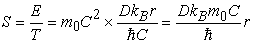(4.3)

This is just the Verlinde entropy gravity proposal formula 5. There is only one constant difference.

We take the formula (4.1) into formula (4.3), we get formula (4.4). It is same as formula (2.1).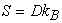(4.4)

So we can found the truth. The Verlinde entropy gravity proposal formula is just a form transformation of mathematical formula. It does not have independent physical meaning. Its physical essence is equivalent to the entropy of a black hole. It is another form of mathematical formula for black hole entropy.

On the other hand, if we assume the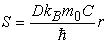at first. Take the condition (4.1) into this formula, we can get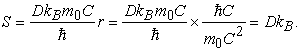This is just the formula (2.1). So the essence of the Verlinde entropy gravity proposal formula is just the formula of the intrinsic entropy of the particle. The entropy in the formula is a constant in fact. If we take the merging condition (4.1) into the intrinsic entropy formula (4.4) of the particle, so we get the Verlinde entropy gravity proposal formula. The entropy gravity formula actually applies only to the particle satisfying the merging condition. It can’t apply to other particles.

We can also use differential formula to represent it. To the particle, it has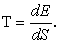So we get formula (4.5).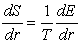(4.5)

Because the energy of particle satisfies formula (4.1). So we get formula (4.6)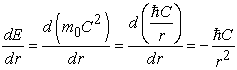(4.6)

The temperature of particle is formula (4.2).

Take (4.2) and (4.6) into (4.5), so we get formula (4.7).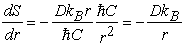(4.7)

Take (4.1) into (4.7) again, so we get formula (4.8).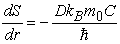(4.8)

Let's take a closer look at this derivation process from (4.5) to (4.8). In the first step, we introduce a basic formula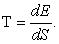But in fact, the differential formula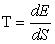cannot be applied to the intrinsic entropy of a particle. To a particle, its intrinsic entropy is formula (4.4). The intrinsic entropy is a constant, so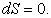So the differential formulacannot be applied to the intrinsic entropy of a particle. Only the formula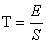can be applied to the intrinsic entropy of a particle. So only the non-differential forms formula (4.3) is correct. The differential forms formula (4.8) is wrong. Please pay attention to this. So the derivation is wrong in author’s previous paper 11. The derivation is just a math game. It does not conform to the principles of actual physics. The author corrects this error here.

On the other hand, let’s look at the formula of the black hole entropy. That is formula (4.9).(4.9)

Formula (4.9) has differential forms.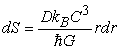(4.10)

To a black hole, there exist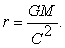M is the total rest mass of the black hole. Take it into formula (4.10), we get formula (4.11).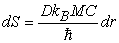(4.11)

So, to a black hole, the differential forms can hold true. But to the particle merging into black hole, the differential forms can’t hold true.

In the derivation process above, the key formula is (4.1). Only particle that satisfy (4.1) can merge with the black hole to form a bigger black hole. Other particles can’t merge with the black hole. The virtual holographic screen is same as a virtual black hole. The process that particles merge into the virtual holographic screen is same as the process of the black hole merging. So, the Verlinde entropy gravity proposal formula only can hold true in the process of black hole merging, and the differential forms of the Verlinde entropy gravity formula is not correct, and only its non-differential forms can hold true.

From above equal temperature condition, we found that the Verlinde entropy gravity proposal formula is not hold true universally. To a black hole with radius r, it is equivalent to a virtual holographic screen with radius r. Only a particle satisfying the condition formula (4.1) can have same temperature with the black hole. We set the rest mass of this special particle by mp.

To these particles which rest mass is more than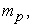its temperature is large than the temperature of black hole. If these particles merge into the black hole, according to thermodynamic principles, the heat will transfer from particle to the black hole, so the temperature of the black hole will increase. This result is conflict with the temperature formula of black hole. From the temperature formula of black hole, the temperature will decrease as the radius of the black hole increases.

To these particles which rest mass is less thanits temperature is small than the temperature of black hole. If these particles merge into the black hole, according to thermodynamic principles, the heat will transfer from the black hole to particles. This result is conflict with the definition of a black hole. From the definition of a black hole, the black hole should capture the outside heat, but not transfer heat to outside.

So we found that whether the particles rest mass is greater than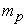or less thanwe will get a conflicting result. So we found that these particles not satisfying the condition formula (4.1) can’t merged into the black hole to form a bigger black hole. Of course, these particles not satisfying the condition formula (4.1) can’t merged into the holographic screen to form a bigger holographic screen.

The particle merge into the holographic screen to form a new object. If we assume the new object is not a holographic screen, so we avoid this contradiction. But this will bring new problem. To the third particle, the new object is not a holographic screen, so the gravity no longer satisfies the Verlinde entropy gravity theory. This is a more serious problem.

So the Verlinde entropy gravity proposal formula does not universally hole true. It is only correct in the process of black hole merging.

But please pay attention carefully. The above discussion can only negate the Verlinde entropy gravity theory, but not all entropy gravity theory. Perhaps there exist other entropy gravity theories that would avoid the conflicts discussed above. The above discussion could prove this result. It is impossible to construct an entropy gravity theory based on black hole entropy. However, the black hole entropy can be seen as a special kind of quantum condensation phenomenon, which can lead to a conclusion. There is some correlation between gravity and entropy.

### 5. Conclusion

We discuss a new black hole merging model in the Planck Gravity theory. The particle only satisfying the merging condition can merge into the black hole to form a bigger black hole. The black hole is a special system of quantum mechanics. The particles have intrinsic entropy in quantum mechanics. The particles arrange together by a special distribution to form a special particles system. The special system is just the black hole. We name the special distribution by black hole distribution. The black hole entropy is just the sum of the intrinsic entropy of these particles merging to form the black hole. We get a conclusion that the black hole entropy is a kind of intrinsic entropy. The black hole temperature is a kind of intrinsic temperature also. The black hole can be seen as a special quantum condensation system, we name it by black hole condensation.

We discuss the physical meaning of this merging condition from multiple perspectives. The condition is equal temperature condition. And the condition is correspondence condition.

Finally, we derive out the Verlinde entropy gravity proposal formula from this black hole model. We proved that the Verlinde entropy gravity proposal formula only hold true in the process of black hole merging. It is not correct universally.

The Planck Gravity Theory is a new quantum gravity theory. There are many topics worth studying in the Planck Gravity Theory. It is hoped that more researchers will join the study of this theory.

### References

  Bekenstein, Jacob D. (April 1973). Black holes and entropy. Physical Review D 7(8): 2333-2346. In article View Article  Bardeen, J. M.; Carter, B.; Hawking, S. W. The four laws of black hole mechanics. Comm. Math. Phys. 31 (1973), no. 2, 161-170. In article View Article  Xiao Lin Li. Planck Gravitation Theory. International Journal of Physics, vol. 7, no. 4 (2019): 118-125. In article View Article  Xiao Lin Li. Empty Hole Entropy and Black Hole Entropy in Gravitation. International Journal of Physics, vol. 6, no. 5 (2018): 155-160. In article View Article  E. P. Verlinde. On the Origin of Gravity and the Laws of Newton. JHEP 1104 (2011) 029 [arXiv:1001.0785 [hep-th]]. In article View Article  David Chandler. Introduction to modern statistical mechanics. ISBN-13: 978-0195042771. In article  R. P. Feynman, R. B. Leighton, M. Sands. The Feynman Lectures on Physics (Volume I, II, III) ISBN 9787506272476, ISBN 9787506272483, ISBN 9787506272490. In article  M. Planck. The Theory of Radiation. Dover 1959 translation, 1906. In article  Sivaram, C. (2007). “What is Special About the Planck Mass?”. https://arxiv.org/abs/0707.0058v1. In article  G.’t Hooft. Dimensional reduction in quantum gravity. arXiv:gr-qc/9310026. In article  Xiao Lin Li. Black Hole Entropy, Verlinde’s Entropy Gravity Proposal and Unruh Formula in Projection Gravitation. International Journal of Physics, vol. 7, no. 2 (2019): 58-62. In article View ArticleThis work is licensed under a Creative Commons Attribution 4.0 International License. To view a copy of this license, visit http://creativecommons.org/licenses/by/4.0/